﻿ Efficient Baseband Digital Predistorter Using Lut for Power Amplifier (PA) with Memory Effect

### Efficient Baseband Digital Predistorter Using Lut for Power Amplifier (PA) with Memory Effect

B. Feuvrie, M. Diop, Y. WangOPEN ACCESSPEER-REVIEWED

## Efficient Baseband Digital Predistorter Using Lut for Power Amplifier (PA) with Memory Effect

B. Feuvrie1, 2, M. Diop3,, Y. Wang1

1IREENA Ecole polytechnique – de l’université de Nantes, Nantes, France

2IUT GEII Nantes - Site Fleuriaye, Av. du Prof J. Rouxel, Carquefou, France

3Ecole Supérieure Polytechnique, UCAD, BP 5085, Dakar-Fann, Sénégal

### Abstract

Modern communications systems will have to integrate multiple radio technologies to support a large number of wireless standards (WLAN, WPAN…). This system should operate with third generation (3G) air interface standards, where non constant envelope modulation formats (MQAM, OFDM…) are required. The amplification of a modulated signal with large variation of envelope introduces distortions when the input-output characteristic is nonlinear. These distortions are particularly important when the power amplifier (PA) has non-linear memory effects with a wideband signal. The technique proposed in this paper aims to fight against this phenomenon by optimizing the method developed in the laboratory for a real-time implementation. This technique is able to provide exactly the reverse characteristics of a PA modeled by a Hammerstein model in base band. Its principle is to find the largest real root of a polynomial related to the analytical model of the PA and to the input signal to be amplified to calculate a pre-distorted signal. To reduce significantly the computation time, an implementation by the LUT method is proposed. This method uses two pre-distortion principles and can be implemented on a FPGA board with table bases method known for simplicity including in the same way the correction of the memory effect.

### At a glance: Figures

• Feuvrie, B., M. Diop, and Y. Wang. "Efficient Baseband Digital Predistorter Using Lut for Power Amplifier (PA) with Memory Effect." American Journal of Electrical and Electronic Engineering 2.3 (2014): 72-81.
• Feuvrie, B. , Diop, M. , & Wang, Y. (2014). Efficient Baseband Digital Predistorter Using Lut for Power Amplifier (PA) with Memory Effect. American Journal of Electrical and Electronic Engineering, 2(3), 72-81.
• Feuvrie, B., M. Diop, and Y. Wang. "Efficient Baseband Digital Predistorter Using Lut for Power Amplifier (PA) with Memory Effect." American Journal of Electrical and Electronic Engineering 2, no. 3 (2014): 72-81.

 Import into BibTeX Import into EndNote Import into RefMan Import into RefWorks

### 1. Introduction

Nonlinear distortions in the transmitter are typically introduced by the RF Power Amplifier (PAs). High efficiency wide-band transmitter design for modern high speed wireless communication systems, such as worldwide interoperability for microwave access (Wimax), third generation and beyond systems, is a complex task. Simultaneously accomplishing high linearity and high power efficiency is a great challenge.

Ideally, the output signal of a PA is proportional to its input signal. But in practice, the output signal is compressed and comes to a saturation zone when the power of the input signal is too high. In this case, the output signal is subjected to the distortions of the amplitude and phase. If the input signal is a modulated signal, two undesirable phenomena can be observed at the output. The first is a distortion of the input signal’s constellation, leading to an increase of the Bit Error Ratio (BER) of the system. The second is the phenomenon of spectral regrowth or the appearance of new spectral components in the adjacent channels. In digital communication systems, adjacent channels may be allocated to the other transmissions and then the interference created (ACI: Adjacent Channel Interference) by nonlinearity becomes unacceptable. The linearity is thus a fundamental characteristic for the design of power amplifiers [1, 2]. One way of suppressing the nonlinear effects is to operate a non linear PA away from its saturation region by adding sufficient back-off to the input signal. However, such amplification schemes possess low-power efficiency since maximum power efficiency is only attained when the PA is operated near its saturation zone. But a good power efficiency is a highly desirable property of a power amplifier, because, it allows to minimize the heat dissipation to reduce the size and the weight of the dissipating elements and thus to increase the autonomy of the terminal. To operate the PAs with high power efficiency, it is necessary to choose an operating point near the compression zone. Under these conditions, we have to correct these undesirable effects by associating, for example, a further PA correction circuit.

Among all linearization techniques, the baseband digital predistortion (DPD) is one of the most cost effective. It is basically relying on the equivalent baseband modeling of the PA and/or its inverse. For digital signal processing convenience, it is very desirable to implement the PD in baseband.

The pre-distortion (PD) is an effective technique which is especially suitable for broadband applications. In order to obtain an output signal proportional to the input signal (Figure 1), the PD technique consists to design a circuit whose characteristics are the opposite to that of PA.

In the Figure 1, the function G represents the nonlinear characteristics of the PA and F those of the PD, which should be the opposite of those the PA. The output y(nT) of the cascade of the two blocks, PD and AP, is written as: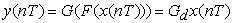(1)

where Gd is a positive constant representing the gain of the linearized amplifier and x(nT) is the input signal. The module and phase of this function must be constant for the whole amplitude range up to saturation.

Different techniques, based on analogue, digital or hybrid circuits, could be adopted while designing the PD. In this paper, we present a technique for baseband adaptive digital pre-distortion (DPD). Its principle is shown in Figure 2. The modulated baseband signal is distorted in DPD, then converted into analog signal, and finally modulated onto RF signal. The pre-distorted signal will be amplified by PA with a linearized output signal.

The baseband DPD is suitable for real-time implementation. In fact, digital circuits working on baseband allow the system reconfiguration, the baseband processing and reduce the sampling constraint which is very important for a modulated signal (often a few GHz). Excellent results on linearity performances can be achieved by the baseband DPD with good power efficiency and flexibility, low costs, and reduced complexity compared to the analogue techniques. The DPD can be implemented on an ASIC (Application-Specific Integrated Circuit) , an FPGA (Field Programmable Gate Array)  or a DSP (Digital Signal Processor) .

In modern SDR (Software-Defined Radio) transmitters, most components must be reconfigurable in order to switch, ideally in the air, from one standard to another. According to these requirements, the DPD technique seems to be an applicable linearization technique. The only limit for the applications of DPD in baseband is the bandwidth of the signal which can be treated by the processor. This limit can be an inconvenient factor for the wide band applications.

In this paper, we present a technique to optimize the technique proposed in . The characteristics of the PA are modeled by a Hammerstein model. This model is a memory less non linearity followed by a linear time invariant (LTI) system.

This model is able to take into account the nonlinearity and also the memory effects of the PA. This method allows to inverse exactly the characteristics of the PA by a direct identification of the behavioral model.

Once the model is identified, the technique corrects the signal perfectly. This method needs to search for the largest real root of a polynomial, which requires too much computation time for a real-time application [17, 20]. In this paper, we propose a technique based on the principle of LUT which will significantly decrease the computation time of the original root-finding based technique.

The paper is organized as follows. The principle of the proposed method is described in Section 2. Section 3 presents the new LUT based technique. The results of the original method and the new method are compared in Section 4. The experimental results are given in Section 5. Finally, a conclusion on the proposed new technique compared to the other existing methods is presented.

### 2. Direct Inversion of the Transfer Function

2.1. Choice of the Method of PD

There are essentially two types of architecture of baseband DPD techniques: the direct and the indirect architectures .

The first type identifies the amplifier model firstly and then reverses it to realize a pre-compensator [14, 15, 16]. From the power amplifier model, it is possible to deduce the value of the output signal of the pre-compensator. The block diagram is presented in Figure 3. This method is chosen in this work. In Section 2.2, it will be discussed in detail.

The second type is based on an indirect identification method (Figure 4) ([8, 9, 10, 13]).

The principle is initially proposed by Gao et al.  to compensate the nonlinearity in the speaker, and then used by Eun et al.  in the DPD with memory.

This class of methods is called indirect method of identification or post-distortion, because the post-inverse of the amplifier is identified and placed before the amplifier . The output signal samples of PA divided by the gain are the inputs of model and the input signal samples of PA are the outputs of model.

The advantage of this identification method is that it can be realized in a single step. Thus, we do not need to concern the modeling of the PA, but directly get its inverse. This greatly facilitates the linearization procedure, even with complex models taking into account the memory effects of the PAs . However, this method has two disadvantages which can affect its performance. The first one is the measurement noise b(n), which appears at the entrance of the identification block. The post-reverse thereby results errors in the identified model. The covariance matrix may be ill-conditioned in some cases. The second one is if the memory of the amplifier is limited, its inverse characteristics will have an infinite memory. Thus some approximations should be made.

2.2. Model of the Power Amplifier

The baseband digital pre-distortion technique with the direct architecture (Figure 3) is chosen in this study.

To model the characteristics of the PA, a Hammerstein model (Figure 5) is used. The static nonlinearity is modeled by a polynomial of odd order (coefficients a2i+1), and the memory effect is represented by a FIR (finite impulse response coefficients hk) filter.

The baseband output signal is written as: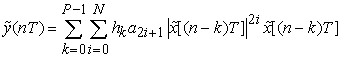(2)

This model can be identified with the least squares method.

2.2. Principle of the Pre-compensator

From the above Hammerstein PA model, it is possible to deduce the value of the output signal of PD. The block diagram of the proposed PD is given in Figure 6:

The PD is composed of two blocks: the direct loop compensates the amplifier nonlinearities and the back loop compensates its memory effect.

The relation between the complex envelopes of the output signal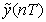and that of the input signal of the power amplifieris given by: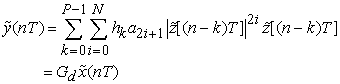(3)

whereis also the pre-distorted signal (output of DPD), Gd is the desired linear gain of the amplifier andis the input signal of DPD representing the signal to be amplified.

Separating the components of the instant and those associated with the previous in formula (3), we get the static part and dynamic part. The static part can be expressed as follows: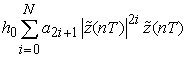(4)

and the dynamic part representing the memory effect is given by: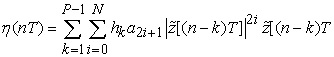(5)

Then, equation (3) can be rewritten as: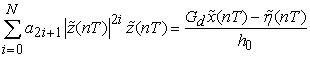(6)

The aim of the system is to find the values ofat the input of the PA to compensate the nonlinearities and the memory effect of the PA. We should solve equation (6) to find. The dynamic part related to the previous values, and the input signal of the system are known at time nT, the amplifier coefficients are identified during the initialization procedure.

The algorithm of the pre-distortion can then be written as:

#### Table 1. PD algorithm

Solving equation (6) can be divided into two parts. Firstly, we should determine the module, which is the largest real and positive root of the following polynomial: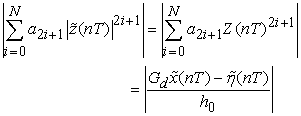(7)(8)

It takes a lot of computer resources and time to calculate the largest real and positive root (theoretically, it is proven that this root always exists [7, 17]).

### 3. Optimization of the above Pre-compensator by Using a LUT

The previous method presents excellent results if the PA model is close to the behavior of the PA. The calculation time is the main problem. Indeed, solving the polynomial equation (7) to determine the root is time-consuming, whichever method (Newton ...) is chosen.

One technique to overcome this handicap is to introduce a table which determines directly the relationship between the input signal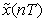and the pre-distorted signal.This technique has the implementation efficiency of the conventional LUT techniques. In addition, it is able to take into account the memory effect without significantly increasing the complexity.

A careful examination of equation (7) shows that the coefficients of the polynomial are constant, only the right member changes for each new sample. This observation allows us to implement a procedure to avoid the time-consuming root-finding procedure. In order to avoid the root searching, we decompose the maximum dynamic rang of the signalby several equal length intervals. Associated with these intervals, the values of the amplitude of the polynomial are calculated by: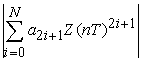(9)

This table provides directly the corresponding the module of the root for each value of the right member of the polynomial (7).

Therefore, for each input, we compute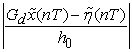to find the corresponding amplitude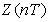and anglegiven in the table. The algorithm is greatly simplified since we only need to calculate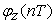by equation (8) andby equation (5). Finally, the new algorithm can be written as given in Table 2.

This new technique achieves almost the same performance as the initial technique described in Table 1. Due to the significant decrease in computation time, the implementation becomes much more feasible. Figure 7 illustrates the main procedures of algorithm.

Nowadays, there are a number of FPGA modules which can produce the DPD by look up tables (they are mostly designed for linearizing memory less nonlinear PAs). The output characteristics of PA are linearized up to the saturation zone. These techniques involve calculating the pre-distorted signal and the corresponding module of the input signal by inversing the input-output characteristic of PA. The values of the input signal are quantified.

In comparison, the proposed method is comprised of a phase calculation process by equation (8) and an operation involving multiplications, divisions and additions by equation (5). These different operations can be easily implemented on FPGAs. The most important point of this technique is that it allows resolving the nonlinearities with memory effect.

### 4. Simulation

4.1. Power Amplifier Model

The PA model aims to simulate the behavior of a PA. The static nonlinearity is modeled by the Saleh model  with coefficients given in Table 3.

The Input-output relation is given by:(10)(11)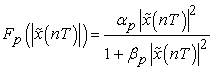(12)

where Fa and Fp are the transfer functions of AM/AM and AM/PM respectively.

AM/AM and AM/PM characteristics are shown in Figure 8. 1 dB compression appears at the average input power of 0.6dBm.

A FIR filter follows the Saleh model to take the memory effect into account. The input-output relationship is given by: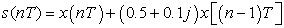(13)
4.2. Comparison of the Two Methods

The above model is used to simulate the behavior of the power amplifier.

The Saleh model with different groups of parameters and M-QAM modulation (M=16) with a raised-cosine filter for pulse shaping (roll-off=0.35) and an up-sampling factor 8 have been adopted to assess the performance.

The symbol rate is 4M symbols/s and 2000 symbols are taken. Initially, the size of the table N is 4096. The output saturation power of the model is approximately 9.75dBm.

The PAPR of a 16QAM signal filtered by a raised cosine filter being about 7.25 dB, the lowest value of output back-off of the linearized PA (LPA) for a possible linearization is OBOmin ≈ 7.25 dB. We can deduce the maximum average output power Plinmax ≈ 2.5dBm corresponding to the input power -3dBm. The values of the EVM and ACPR for various levels of the output power are shown in Figure 9.

The power spectral density (PSD) of input/output signals of the PA is estimated using the Welch method in Matlab. The length of each segment is 300 samples, with 50% overlap between each segment (one hundred segments totally). A window of Blackman is used. The Matlab function which performs this calculation is the function “psd (•)”. The use of the Blackman window minimizes the side lobe level due to the finite length of data segments. This is very important for precisely estimating the power spectral density (PSD) of the linearized and non linearized spectrums which have an important dynamic. Lets note that other types of window can be used with results comparable to those obtained with the Blackman window (Han, Chebyshev, Parzen, Bartlett, Hanning, etc...). This method of estimating the PSD of the signals is also adopted in real measurement, presented later.

We can see that with an output power of 2.5dBm, the performance, in terms of ACPR, degrades rapidly. The speed of degradation depends on the proportion of samples which enter the saturation zone.

A significant improvement of ACPR has been observed when the average output power does not exceed 2.5dBm in Figure 9 (a) with a maximum correction of 19dB.

The memory effect is well corrected in the operating zone (2,5dBm). Without pre-distortion, in the linear zone (with low input power), we can observe a residual EVM due to the memory effect from Figure 9 (b). We can observe also that for an average output power around 3dBm and 5dBm, the pre-distortion becomes less effective for ACPR and EVM respectively.

The performances deteriorate rapidly with the method of direct polynomial root searching, because the identified model becomes less precise for the samples of high power. But with the LUT based method, the degradation is slower. As the average output power increases, the larger the samples deviate from the model, the more the errors caused by the root finding technique.

Figure 11. a- Polynomial identified model (solid line: identified model; discrete lines: samples of PA, Average power input -2dBm); b- Polynomial identified model (solid line: identified model; discrete lines : samples of PA, Average power input 0dBm)

Figure 10 and Figure 11 show that when the power of input signal increases, the gap between the samples of PA output signal and the samples of the identified model increases.

In the following, to analyze the influences of different parameters, we set the input power equal to -5dBm. The identification of the Hammerstein model is achieved by the least squares method. The simulation results are presented as follows with the table size N of 4096.

The eye diagram of the nonlinear output signal in Figure 12 (b) is strongly distorted compared with the eye diagram of the input signal Figure 12(a).

Constellations of input signal and the output signal without linearization are presented in Figure 13. Rotations and dispersions of constellations are due to the nonlinearity of PA. The results with linearization are shown in Figure 14. Perfect reshaping symbols are achieved.

Figure 13. a- Constellation of the input signal, (EVM=0%); b- Constellation of output signal without linearization (EVM=17.53%)
Figure 14. a- Constellation with linearization, Method with root calculation (EVM=0.44%); b- Constellation with linearization, Method with LUT (EVM=0.44%)

In Figure 15, a significant improvement on the regrowth spectrum is observed by the proposed LUT based method which is almost identical to the method based on direct root finding of a polynomial.

The ACPR is resume in table

4.3. Influence of the Size of the Table

A simulation with a table size of 64 has been made and compared with the table size of 4096 Figure 16.

There is a residual distortion of the constellation after linearization with a table size N of 64. This is due to the quantization effect on the pre-distorted signal. But the EVM still has a very low value.

Figure 17 presents a level of -52dBm in the adjacent channel for the output of the PA without linearization. Compared with the method based on the polynomial root finding, the proposed method achieves an ACPR of -49.6 dB instead of -59.6 dB. If the table size is increased to 256, the ACPR will be of -59 dB and the EVM of 0.8%.

The proposed method is more appropriate for a real time implementation. Of course, it has the same limitation as all the linearization techniques: the average power of input signal should not be too close to the saturation zone. In these conditions, according to the value of the PAPR, the quality of PD will decrease sharply.

### 5. Experimental Results

5.1. Test Bench Description

In this section, we first describe the test bench designed for the experiments. Then, we will evaluate the performance of the digital baseband pre-distortion technique, by using an amplifier from Mini-Circuits, ZFL-2500, driven by a 16-QAM modulated signal.

In order to evaluate the proposed linearization techniques, we begin by identifying a model of the PA from the input/output signals from the power amplifier under test. Then, we present the experimental results, and compare them to the theoretical ones.

The performance of the PD is evaluated by measuring two important parameters, Adjacent Channel Power Ratio (ACPR) and Error Vector Magnitude (EVM), with different values of power output signal.

The test bench  consists of a Vector Signal Generator (VSG), a digital oscilloscope (DO) and a personal computer (PC) (Figure 18). It is designed to be fully automatic by using Matlab toolbox. The data are generated in Matlab and then sent to the VSG. The VSG (Rhode & Schwartz SMU 200A) receives the complex envelope data via an Ethernet cable (TCP/IP) from the PC and uses a direct up-conversion from baseband to RF, produces virtually any signal within its bandwidth limits. Note that, once the data have been sent to the VSG, the latter will repeatedly send the corresponding modulated signal to the PA. A marker can be activated to trigger the DO each time when the sequence is regenerated.

The RF input and output signals of the PA are then sampled simultaneously in the real time oscilloscope (Leroy, 4 channels Wave master 8600, 6GHz bandwidth, 20 GS/sec), and then transferred via an Ethernet cable to the PC, and finally recorded in the of workspace Matlab.

The acquisition time in the DO is fixed to be equal to the duration of the baseband signal generated by Matlab. In this way, the acquired RF signals correspond exactly to the original signal of Matlab. After that, the two sequences are digitally demodulated in Matlab, in order to identify the parameters and evaluation can be implemented in a single program running in Matlab without interruption. Note that, for signal acquisition, the spectrum analyzer “Agilent E4440A” can also be used as an alternative tool for precision, comparison and verification. In this case, the signal analysis software provided with this instrument can be used to demodulate and acquire the input and output signals separately.

The signals can be synchronized by correlating them with the original of Matlab signal.

After that, the two sequences are digitally demodulated in Matlab, in order to identify the parameters of the PD. The baseband signal is then processed by the pre-distortion function and loaded again to the VSG. Finally, the output of the linearized PA is digitized in the DO and sent back to the PC to evaluate the performance of the PD. This evaluation can be done by comparing the output spectrum (ACPR) and constellation distortion (EVM) of the PA with and without linearization at different back-off levels.

The time cost of this entire test is just several minutes since this test bench is fully automatic. In other words, the signal transmission, acquisition, identification and evaluation can be implemented in a single program running in Matlab without interruption.

Note that, for signal acquisition, the spectrum analyzer “Agilent E4440A” can also be used as an alternative tool for precision, comparison and verification. In this case, the signal analysis software provided with this instrument can be used to demodulate and acquire the input and output signals separately.

The signals can be synchronized by correlating them with the original of Matlab signal.

Measurements have been carried out on an amplifier ZFL 2500 from Mini-circuits. This wide-band (500-2500 MHz) PA is used in several types of application, typically in GPS and cellular base stations. According to its data sheet, it has a typical output power of 15dBm at a 1 dB compression point, and a small signal gain of 28 dB (±1.5).

In order to validate the study presented in Section 3, we have performed the one tone test on this PA.

The defined parameters, namely, compression, interception and saturation points are also very useful for the experimental evaluation of the DPD technique. Figure 19 shows the AM/AM characteristic of the PA under test.

In order to compare the two methods, we use a modulated 16 QAM signal. The pulse shaping filters are raised cosine filters with a roll-off factor 0.35 extending 4 symbols on either side from the centre and 20 times oversampled. The carrier frequency is 1.8GHz and the bandwidth is 4MHz.

The parameters of the modulated signal are presented in Table 5:

For a precise comparison, we will do the same measurements to evaluate the method based on the polynomial root finding. The results presented below are obtained with the following parameters:

•  5 coefficients NL (Length of non-Linearity)

•  2 coefficients EM (Memory effect depth)

•  1024 coefficient N (Size of table)

After the identification of the Hammerstein model coefficients, the pre-distorted signal is determined by the LUT based method and then re-injected into the amplifier.

Note that the output saturation power Pssat of ZFL-2500 is approximate to 19.7dBm. Knowing that the PAPR of the modulated 16QAM signal filtered by raised cosine is approximate to 7.25dB, and the lowest value of output back-off of the linearized PA (LPA) for a possible linearization is 7.25dB for OBOmin, with Plinmax of 12.45dBm. It is important to note that the probability density of the 16QAM signal amplitude and its PAPR, changing with the filter, no matter raised cosine (CS) or root raised cosine (SCR) is adopted.

In fact, the performances of PD by the two methods are very similar. The maximum correction is very close to the maximum linear power, Plinmax, approximately from the average output power of 12.2 dBm for ACPR and EVM to 14dBm. In Figure 20, the correction for the ACPR is just 10dB. This is due to the fact that DO does not present a sufficient quantization of the input signal (8 bits). The precision of the DSP cannot go low enough. For the EVM, the improvement is 3%. The difference between measurement and simulation is the unavoidable noise, especially the phase noise.

However, the PD by root solving and the exact optimized method by table reach nearly the same performance throughout the power range, especially near Plinmax.

### 6. Conclusion

The method proposed in this paper has two substantial advantages compared to the original method.

First, the original method proposed in  taking into account the memory effects becomes implementable on a FPGA. But the algorithm proposed in this paper contains only simple operation (additions, multiplications), better suited for real-time implementation. The complex step of polynomial root solving is removed.

Secondly, this method improves performances for the high power levels of input signal. In fact, the divergence of the polynomial model deteriorates the performance of PD before a certain average power in the non-linear zone, where the input signal samples enter the different zones. The implementation by table solves this problem.

As soon as the level of the input signal becomes large enough, the pre-distorted samples selected correspond to the highest level in the table. They are independent on the model coefficients if the dynamic parts of the signal for filling the table are properly chosen.

The proposed method retains the advantages of the LUT method by which correcting the memory effect becomes easier.

For a perfectly linearized amplifier, it must introduce a minor decrease (back-off) which is equal to the PAPR of the input signal. With a smaller OBOmin, the spectral regrowth increases very quickly.

Thus it would be important to study the existing techniques for reducing the PAPR of the modulated signals, associated with the pre-distortion technique.

### References

  P. B. Kenington, “High Linearity RF Amplifier Design”, Norwood, MA, USA: Artech House, Inc., 2000.In article  S. C. Cripps, “RF Power Amplifiers for Wireless Communications”, Second Edition (Artech House Microwave Library (Hardcover)), Norwood, MA, USA: Artech, House, Inc., 2006.In article  L. Sundstrom, M. Faulkner, and M. Johansson, “Quantization analysis and design of a digital predistortion linearizer for RF power amplifiers”, IEEE Transactions on Vehicular Technology, vol. 45, no. 4, pp. 707-719, Nov 1996.In article CrossRef  N. Safari, J. Tanem, and T. Roste, “A block-based predistortion for high poweramplifier linearization”, IEEE Transactions on Microwave Theory and Techniques, vol. 54, no. 6, pp. 2813-2820, June 2006.In article CrossRef  M. Faulkner and M. Johansson, “Adaptive linearization using predistortion experimental results”, IEEE Transactions on Vehicular Technology, vol. 43, no. 2, pp. 323-332, May 1994.In article CrossRef  W.-J. Kim, S. Stapleton, J. H. Kim, and C. Edelman, “Digital predistortion linearized wireless power amplifiers”, IEEE Microwave Magazine, vol. 6, no. 3, pp. 54-61, Sept. 2005.In article CrossRef  Cottais, E.; Nomm, S.; Wang, Y.; Toutain, S.; Moog, C. “Baseband adaptive predistorter to linearize power amplifiers with memory”, European Microwave Conference, Amsterdam, Netherlands, October 2004.In article  D. Morgan, Z. Ma, J. Kim, M. Zierdt, and J. Pastalan, “A generalized memory polynomial model for digital predistortion of rf power amplifiers”, IEEE Transactions on Signal Processing, vol. 54, no. 10, pp. 3852-3860, Oct. 2006.In article CrossRef  T. Liu, S. Boumaiza, and F. Ghannouchi, “Augmented hammerstein predistorter for linearization of broad-band wireless transmitters”, IEEE Transactions on Microwave Theory and Techniques, vol. 54, no. 4, pp. 1340-1349, June 2006.In article CrossRef  L. Ding, G. Zhou, D. Morgan, Z. Ma, J. Kenney, J. Kim, and C. Giardina, “A robust digital baseband predistorter constructed using memory polynomials”, IEEE Transactions on Communications, vol. 52, no. 1, pp. 159-165, Jan. 2004.In article CrossRef  F. Gao and W. Snelgrove, “Adaptive linearization of a loudspeaker”, International Conference on Acoustics, Speech, and Signal Processing, 1991 (ICASSP1991), pp. 3589-3592 vol.5, Apr 1991.In article  C. Eun and E. Powers, “A new Volterra predistorter based on the indirect learning architecture”, IEEE Transactions on Signal Processing, vol. 45, no. 1, pp. 223-227, Jan 1997.In article CrossRef  Marsalek, R.; Jardin, P. & Baudoin, G., “From post-distorsion to pre-distorsion for power amplifiers linearization”, IEEE Communications Letters, Vol. 7, pp. 308-310, 2003.In article CrossRef  M. Schetzen, “The Volterra and Wiener Theories of Nonlinear Systems”, Melbourne, FL, USA: Krieger Publishing Co., Inc., 2006.In article  Y. H. Lim, Y. S. Cho, I. W. Cha, and D. H. Youn, “An adaptive nonlinear prefilter for compensation of distortion in nonlinear systems”, IEEE Transactions on Signal Processing, vol. 46, no. 6, pp. 1726-1730, Jun 1998.In article CrossRef  H. W. Kang, Y. S. Cho, and D. H. Youn, “On compensating nonlinear distortions of an ofdm system using an efficient adaptive predistorter”, IEEE Transactions on Communications, vol. 47, no. 4, pp. 522-526, Apr 1999.In article CrossRef  E.Cottais, “Linéarisation d’amplificateurs de puissance large bande par pré-distorsion adaptative en bande de base”, Ph.D.dissertation, Université de Nantes – France, 5 Dec 2005.In article  M. Abi Hussein, “Linéarisation des amplificateurs de puissance – Pré-distorsion numérique adaptative en bande de base”, Ph.D.dissertation, Université de Nantes – France, 3 Jun 2009.In article  R.Marsalek, “Contribution à la linéarisation des amplificateurs de puissance par prédistorsion numérique adaptative en bande de base”, Ph.D.dissertation, ESSIE NOISY LE GRAND– France, 12 Dec 2003.In article  E.Cottais, Y.Wang, S.Toutain, “A new adaptive baseband digital predistortion technique”, European Microwave Association, vol. 2, juin 2006, pp 154-159.In article  A. Saleh, “Frequency-independent and frequency-dependent nonlinear models of twt amplifiers,” Communications, IEEE Transactions on, vol. 29, no. 11, pp. 1715-1720, Nov 1981.In article CrossRef